# Removing Thermal Offset Errors from Contact, Switch, and Relay Resistance Measurements Tutorial

Publish Date: Feb 03, 2017 | 14 Ratings | 3.21 out of 5 | Print | Submit your review

## Overview

Automated test systems are used in industries ranging from semiconductors to communications (cellular phones) to automotive (brake systems). Most of these systems use some kind of switching to increase test points. Certain common types of switches can introduce thermal voltage offsets into circuit measurements because of the materials used in their construction. Engineers have traditionally dealt with this problem by either approximating the error or taking additional measurements. Either method can cause slower test system throughput or increased cost. New technologies are reducing the effect of thermal offset and bringing additional benefits including:
• Simplified test sequences and test system architecture
• Increased automated test throughput and system utilization, resulting in cost reductions
• Increased accuracy, resulting in more correct pass/fail final system verifications
This document will describe the traditional methods and it will describe how to use the NI 4070 FlexDMM Offset Compensated Ohms (OCO) feature to remove thermal offset errors from low-level contact, switch, and relay resistance measurements. You can also view an interactive presentation that takes you through this tutorial's material at your own pace.

For additional information and more interactive tutorials on the NI 4070, visit the Digital Multimeter Measurement Tutorial.

For product information on NI digital multimeters and switches please see:
National Instruments Digital Multimeter (DMM) and LCR Meter
National Instruments Switches

Low-Level Measurement Resources

### 1. Low-Level Measurement Setup

The best setup for low resistance measurements is the 4-wire setup. A 4-wire setup utilizes four leads, two leads to drive current through a resistance and two leads to measure the voltage drop across that resistance. Traditional 4-wire measurements require that the power is off in the circuit so that the measured voltage drop is only due to the supplied current. Thus traditionally, the power to a circuit must be turned off before any measurements are taken. Figure 1 below illustrates a 4-wire setup. The black-colored leads measure the voltage drop. The blue-colored leads connect the test current, which is 1 mA in the 100 ohm range.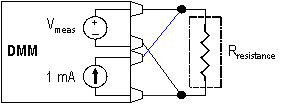Figure 1: Digital Multimeter Setup

In every resistance measurement, the digital multimeter must supply a test current, 1 mA in this setup, and then measure the voltage drop across the unit under test. The measured voltage drop is then used in the calculation for resistance, thus its accuracy is very important. This accuracy can be negatively affected by thermal offsets in switches or relays. This tutorial discusses possible sources of error in four measurement methods.

The four comparison measurement methods are:

1. No Compensation
2. Approximated Offset
3. Measured Offset

### 2. Reed Relay Contact Resistance

A typical contact resistance measurement application is measuring the contact resistance on a relay or switch as shown in Figure 2. A dry reed relay can have a contact resistance between 0.1 and 2 ohms. For this example, we can assume that our relay (Rreed relay) has a resistance of 0.1 ohms.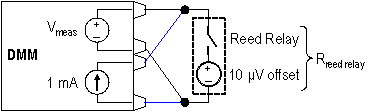Figure 2: Reed Relay Contact Resistance Measurement

### 3. Case 1: No Compensation

In this reed relay system, the switch lead material has a thermal offset which can affect the accuracy of the measurement. Uncompensated reed relays can have offset voltages greater than 10 uV caused by the Kovar lead material used at the device glass seal. This offset can dramatically affect a low-level resistance measurement.

The lead material offset is represented in the schematic in Figure 2 by a 10 uV source in-line with the reed relay. Therefore, the measured voltage drop on the digital multimeter will include the voltage across the reed relay as well as the 10 uV offset. When the reed relay’s resistance is calculated it will also include the offset, giving an incorrect reading.

The voltage that is measured by the digital multimeter is equal to the offset voltage plus the test current times the resistance of the reed relay. In this case, that measurement would be 10 uV, from the lead offset, plus 1 mA times the contact resistance of the reed relay. Since the resistance of our reed relay is 0.10 ohms, the measured voltage will be 0.11 mV.

Vmeas = Offset Voltage + Test Current x Rreed relay
Vmeas = 10 uV + 1 mA x Rreed relay
Vmeas = 0.11 mV

The calculated or measured resistance then is equal to the measured voltage divided by the test current. In this case, the measured resistance is 0.11 ohms. This is an error of 10% from the actual resistance of 0.10 ohms.

Rmeas = Vmeas / Test Current
Rmeas = 0.11 mV / 1 mA
Rmeas = 0.11 ohms
Error = (0.11 ohms - 0.10 ohms) / 0.10 ohms
Error = 10%

This error may be unacceptable in certain applications, and there are various methods to reduce the error.

View the interactive presentation

### 4. Case 2: Approximated Offset

To get an accurate resistance measurement, the lead material offset must be removed from the measurement. One way to do this is to approximate the offset error based on the type of junction in your reed relay. A few sample junction types are shown in the table below.

 Junction Type Potential EMF Cu-Kovar 40 uV/°C Cu-Si 400 uV/°C Cu-CuO 1000 uV/°C

There are two drawbacks to this type of adjustment. First, an external current excitation source must be used since voltage, not resistance, must be measured. The digital multimeter setup is shown in Figure 3 below.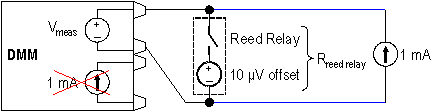Figure 3: Digital Multimeter Setup for voltage measurement with external current supply

Second, additional calculations must be done by hand to obtain resistance. The measured voltage would still include the actual offset voltage, but when calculating the resistance, an estimated offset could be removed. The major disadvantage to this method is that the actual offset will not be known.

Vmeas = Offset Voltage + Test Current x Rreed relay
Rcalc = (Vmeas - Estimated Offset) / Test Current

View the interactive presentation

### 5. Case 3: Measured Offset

Another method overcomes this disadvantage, by measuring the actual offset voltage and then taking it out of the resistance calculation. With a traditional digital multimeter the drawbacks include:

• Multiple voltage measurements
• An external current excitation source
• Additional calculations that must be done by hand

The same reed relay resistance application using the measured offset technique would require the following steps.

1. Take a voltage measurement with an external current supply (as shown in Figure 3)
2. Take a second voltage measurement with no current excitation as shown in Figure 4 below. This measurement will effectively measure the offset in the system.
3. Use the difference of the voltages to calculate resistance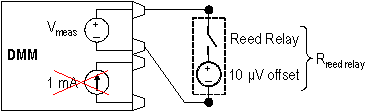Figure 4: Digital Multimeter Setup for voltage measurement with no current excitation

The calculations for this method are as follows. The voltage drop across the reed relay is again equal to 10 uV, from the lead offset, plus 1 mA times the contact resistance of the reed relay. The measured voltage of 0.11 mV is the same as in the previous traditional non-compensated measurement.

Vmeas #1 = Offset Voltage + Test Current x Rreed relay
Vmeas #1 = 10 uV + 1 mA x Rreed relay
Vmeas #1 = 0.11 mV

With the second measurement using the measured offset technique, the 1 mA test current is not used, and so the measured voltage changes. The voltage drop across the relay drops to zero because no current is flowing through it and thus only the value of the 10 uV offset is measured.

Vmeas #2 = Offset Voltage
Vmeas #2 = 10 uV

The difference in the two measured voltages represents the actual voltage drop across the reed relay. This voltage is divided by the test current to calculate an accurate resistance for the reed relay. Thus any error due to the lead material offset is removed.

Vcalc = Vmeas #1 - Vmeas #2
Vcalc = 0.11 mV - 10 uV
Vcalc = 0.10 mV

Rmeas = Vcalc / Test Current
Rmeas = 0.10 mV / 1 mA
Rmeas = 0.10 ohms

### 6. Case 4: Offset Compensated Ohms (OCO)

A final method incorporates the accuracy of the measured offset method but maintains the simplicity of the no compensation technique. This method, called Offset Compensated Ohms (OCO) is available on the NI 4070 FlexDMM. It overcomes the requirements of multiple voltage measurements, external current excitation sources, and additional calculations, yet is still able to measure the actual offset voltage and take it out of the resistance measurement.

Once enabled and without any additional user interaction, Offset Compensated Ohms invokes the NI 4070 FlexDMM to take two resistance measurements, but with the test current source off during the second measurement. The first measurement includes the voltage drop across the relay and any thermal EMFs that may be present as shown in Figure 5.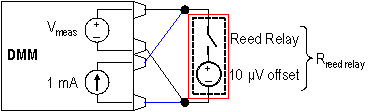Figure 5: NI 4070 FlexDMM - 1st OCO Measurement with internal excitation ON

During the second measurement the current source is turned off so the voltage drop across the relay becomes zero volts. This second measurement only measures the thermal offset as shown in Figure 6.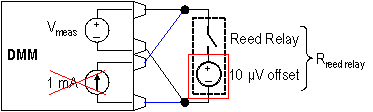Figure 6: NI 4070 FlexDMM - 2nd OCO measurement with internal excitation OFF

The NI FlexDMM then subtracts the second measurement from the first to determine the Offset Compensated voltage (VOCO). This voltage is then used to determine the correct resistance value.

VOCO = Vmeas #1 - Vmeas #2
VOCO = 0.11 mV - 10 uV
VOCO = 0.10 mV

Rmeas = VOCO / Test Current
Rmeas = 0.10 mV / 1 mA
Rmeas = 0.10 ohms

### 7. Summary

The chart below is a comparison of the methods to improve the accuracy of low-level, contact resistance measurements including the Offset Compensated Ohms technique on the NI 4070 FlexDMM.Case 1: No Compensation Case 2: Approximated Offset Case 3: Measured Offset Case 4: Offset Compensated Ohms (OCO) Number of Measurements 1 1 2 1 External Current Supply No Yes Yes No Accuracy OK Better Best Best Possible Error 10% less than 10% Negligible Negligible Sources of Error No compensation Bad estimate No procedural error No procedural error Complexity Easy Moderate Difficult Easy (no setup changes)

### Ratings

Rate this document